# Hydrostatic Pressure

## Function

• Enter the hydrostatic pressure (fluid potential) loads at the edges or faces of Plate, Plane Stress, Plane Strain or Solid Elements. The loads can be specified as linear loads or curved loads. Curved loads can be used for earth pressure (such as due to earthquakes) acting on underground structures.
• The hydrostatic pressure loads are calculated at each corner node of the elements. The point pressure is obtained by multiplying the distance from the given surface of the fluid by the density of the fluid.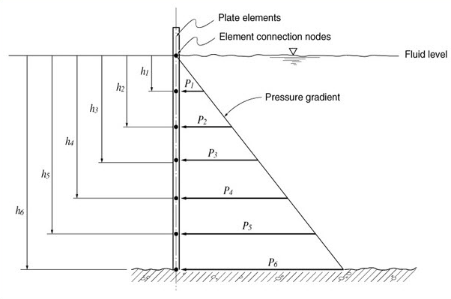Pressure loads due to the fluid potential at the connection nodes of plate elements

## Call

From main menu, select [Load] tab > [Type : Static Loads] > [Pressure Load] group >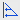[Hydrostatic Pressure]

## Input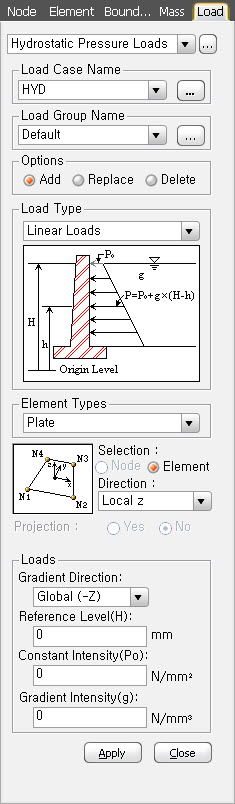Assign the load case name. Click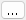to the right to enter additional load cases and modify or delete existing load cases.

Select the desire Load Group that will include the entered Hydrostatic Pressure Loads data. Select "Default", if a Group assignment is unnecessary. Clickto the right to add, modify or delete Load Groups.

### Options

Replace : Replace previously entered hydrostatic pressures

Delete : Delete previously entered hydrostatic pressures

Load Type of pressure load based on the potential of fluid is specified.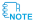Curved Loads are loaded on three equally divided segments of elements. If an element is long, the load distribution becomes close to a straight line. So the elements need to be sufficiently divided to closely represent curved load distribution.

### Element Type

Select the type of elements to be subjected to pressure loads. Refer to the figure at the bottom to assign the loading direction and the loaded side(s).

Plate : Plate element

Section : Select loaded objects by either nodes or elements

Direction

Local x : Pressure loads applied in the element local x-direction

Local y : Pressure loads applied in the element local y-direction

Local z : Pressure loads applied in the element local z-direction

Global X : Pressure loads applied in GCS X-direction

Global Y : Pressure loads applied in GCS Y-direction

Global Z : Pressure loads applied in GCS Z-direction

Plane Strain : Plane strain element

Axisymmetric : Axisymmetric element

Pressure Edge : Refer to the figure to select the edge numbers to be loaded.

8 Nodes Solid : Solid element 8 Nodes Solid

6 Nodes Solid

4 Nodes Solid

Pressure Face : Refer to the figure to select the face number to be loaded.

Direction

Normal : Pressure loads applied normally to the face of the solid elements

Global X : Pressure loads applied in GCS X-direction

Global Y : Pressure loads applied in GCS Y-direction

Global Z : Pressure loads applied in GCS Z-direction

Projection

When the pressure loads are applied to plate or solid elements in the direction of 'Global X, Y or Z', select whether or not to project the loads on a plane perpendicular to the loading direction.

Yes : Project the pressure loads

No : Pressure loads are applied along the entire face

The application conditions for hydrostatic pressure loads are as follows:

Hydrostatic Pressure = P0 + g(H - h)

Where, H > h (position of the element connection nodes)

Gradient Direction : Assign the gradient direction of the hydraulic potential - increasing direction from the fluid surface

When Gradient Direction is Global(-X) : The negative (-) direction of the X-axis in the global coordinate system of a fluid surface

When Gradient Direction is Global(-Y) : The negative (-) direction of the Y-axis in the global coordinate system of a fluid surface

When Gradient Direction is Global(-Z) : The negative (-) direction of the Z-axis in the global coordinate system of a fluid surface

Reference Level(H) : Reference level for the pressure due to the hydraulic potential of fluids (enter with the mouse or keyboard)

Constant Intensity(P0) : Pressure acting on the fluid surface

Gradient Intensity(g) : Specific weight of fluidIn order to apply lateral soil pressure onto a structure as Hydrostatic Pressure Loads, the following needs to be observed:

Element Type needs to the Plate type. The structure should be divided into small elements so that its flexural behavior is well reflected. The Direction is defined as the direction of the acting loads. Gradient Direction is generally specified as the gravity direction (Global-Z). Constant Intensity (Po) is entered to reflect the soil pressure due to overburden to which the soil pressure coefficient is applied. Gradient Intensity (g) is applied with the soil pressure coefficient and entered as follows depending on whether or not the level of ground water is considered.

1) When ground water level is not considered (only soil effect is entered)

Soil : g = soil pressure coefficient * unit weight of soil

2) When ground water level is considered (effects due to soil and ground water are separately entered)

Soil: g = soil pressure coefficient * unit weight of submerged soil

Ground water: g = unit weight of water

(For ground water, Reference level (H) is the ground water level.)

The application conditions for hydrostatic pressure loads are as follows:

Gradient Direction : To specify the cumulative direction of pressure due to the potential energy of a fluid

When Gradient Direction is Global(-X) : The negative (-) direction of the X-axis in the global coordinate system of a fluid surface

When Gradient Direction is Global(-Y) : The negative (-) direction of the Y-axis in the global coordinate system of a fluid surface

When Gradient Direction is Global(-Z) : The negative (-) direction of the Z-axis in the global coordinate system of a fluid surface

Reference Level(H) : Reference level for the pressure due to the hydraulic potential of fluids (enter with the mouse or keyboard)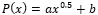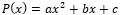a, b, c : To input the coefficient values of a polynomial function or a quadratic function

0